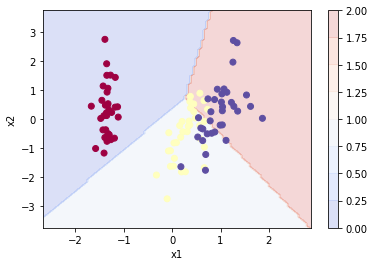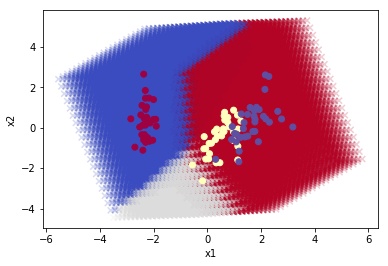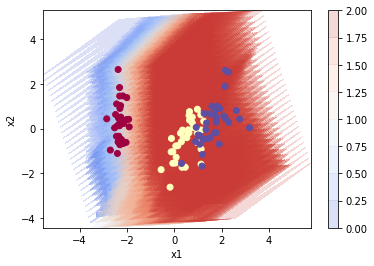# Visualizing High Dimensional Data¶

### Elan Ding¶

Modified: July 18, 2018

This post is to show some custom functions that I wrote over the past week. The package named elan can be found on Github here. My goal is to visualize a high dimensional data set in 2D and 3D, and also plot the decision boundaries for any given classification model. Let's call these libraries first.

In :
import numpy as np
import pandas as pd
from elan.DL import *
from elan.ML import *


For testing purpose, we use the famous Iris dataset. We split the data frame into data and label, where data is a matrix of dimension $(m, n_x)$ where $m$ is the number of training examples and $n_x$ is the number of features. The label vector has dimension $(1, m)$.

In :
df = pd.read_csv("IRIS.csv")

data = np.array(df.iloc[:,:4])
data = standardize(data)

label = np.array(df.iloc[:,-1])

# Change categorical values to numeric
label_encode = encode(label)


Next we project the feature space onto its first two principal components using the custom function pca_transform.

In :
data_pca, _ = pca_transform(data)


Also, for simplicity, we train two models using this data. The first model is a logistic regression fitted through the two principal components, and the second model is the usual logistic regression fitted throught the original data.

In :
model_pca, _ = glm(data_pca, label_encode)
model, _ = glm(standardize(data), label_encode)

The 5-fold cross validation score is (0.6164943358888114, 1.0704463051694768)
The 5-fold cross validation score is (0.7219647765545357, 1.0571878355392526)


For added bonus, check out the function pca_plotly that I wrote. This function is incredibly easy to use. All you need to do is to specify your data, label, and the dimension, which can be either 2 or 3.

pca_plotly(data, label, 2)

pca_plotly(data, label, 3)


Next it is time to view some decision boundaries. First we look at the decision boundary of the logistic regression fitted through the compressed data. By the way, I wrote two versions. The first one pca_contour uses contour plots, so it will be much smoother. The second one pca_scatter first divides the feature space into grids and use scatter plots to display the regions of classificaion.

In :
pca_contour(model_pca.predict, data_pca, label_encode, 100)

Assuming that model is fit with standardized data.
Model must give the same output as the label.In :
pca_scatter(model.predict, data, label_encode, 20)

Assuming that model is fit with standardized data.What about high dimensional data? So let's take a look at the decision boundary of a logistic regression through the original data set (which has dimension 4).

In :
pca_contour(model.predict, data, label_encode, 30)

Assuming that model is fit with standardized data.
Model must give the same output as the label.How beautiful! I was absolute stunned by the beauty after my code worked. This plots the projection of a 4-dimensional decision boundary onto the plane! Incredible. Let's take a look at a different flavor.

In :
pca_scatter(model.predict, data, label_encode, 20)

Assuming that model is fit with standardized data.Looking at these plots makes me realize the complexity of high dimensions. They cannot be visualized, yet we can learn so much about them using mathematics.